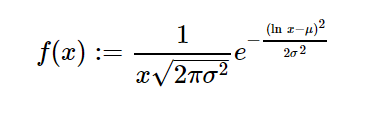# sympy.stats.LogNormal() in python

With the help of `sympy.stats.LogNormal()` method, we can get the continuous random variable which represents the Log-Normal distribution.Syntax : `sympy.stats.LogNormal(name, mean, std)`
Where, mean and standard deviation are real number.
Return : Return the continuous random variable.

Example #1 :
In this example we can see that by using `sympy.stats.LogNormal()` method, we are able to get the continuous random variable representing Log-Normal distribution by using this method.

 `# Import sympy and LogNormal ` `from` `sympy.stats ``import` `LogNormal, density ` `from` `sympy ``import` `Symbol, pprint ` ` `  `z ``=` `Symbol(``"z"``) ` `mean ``=` `Symbol(``"mean"``, positive ``=` `True``) ` `std ``=` `Symbol(``"std"``, positive ``=` `True``) ` ` `  `# Using sympy.stats.LogNormal() method ` `X ``=` `LogNormal(``"x"``, mean, std) ` `gfg ``=` `density(X)(z) ` ` `  `pprint(gfg) `

Output :

2
-(-mean + log(z))
——————-
2
___ 2*std
\/ 2 *e
————————–
____
2*\/ pi *std*z

Example #2 :

 `# Import sympy and LogNormal ` `from` `sympy.stats ``import` `LogNormal, density ` `from` `sympy ``import` `Symbol, pprint ` ` `  `z ``=` `2.1` `mean ``=` `7.6` `std ``=` `4` ` `  `# Using sympy.stats.LogNormal() method ` `X ``=` `LogNormal(``"x"``, mean, std) ` `gfg ``=` `density(X)(z) ` ` `  `pprint(gfg) `

Output :

0.0136890249307238*\/ 2
————————
____
\/ pi

Attention geek! Strengthen your foundations with the Python Programming Foundation Course and learn the basics.

To begin with, your interview preparations Enhance your Data Structures concepts with the Python DS Course.

My Personal Notes arrow_drop_upCheck out this Author's contributed articles.

If you like GeeksforGeeks and would like to contribute, you can also write an article using contribute.geeksforgeeks.org or mail your article to contribute@geeksforgeeks.org. See your article appearing on the GeeksforGeeks main page and help other Geeks.

Please Improve this article if you find anything incorrect by clicking on the "Improve Article" button below.

Article Tags :

Be the First to upvote.

Please write to us at contribute@geeksforgeeks.org to report any issue with the above content.